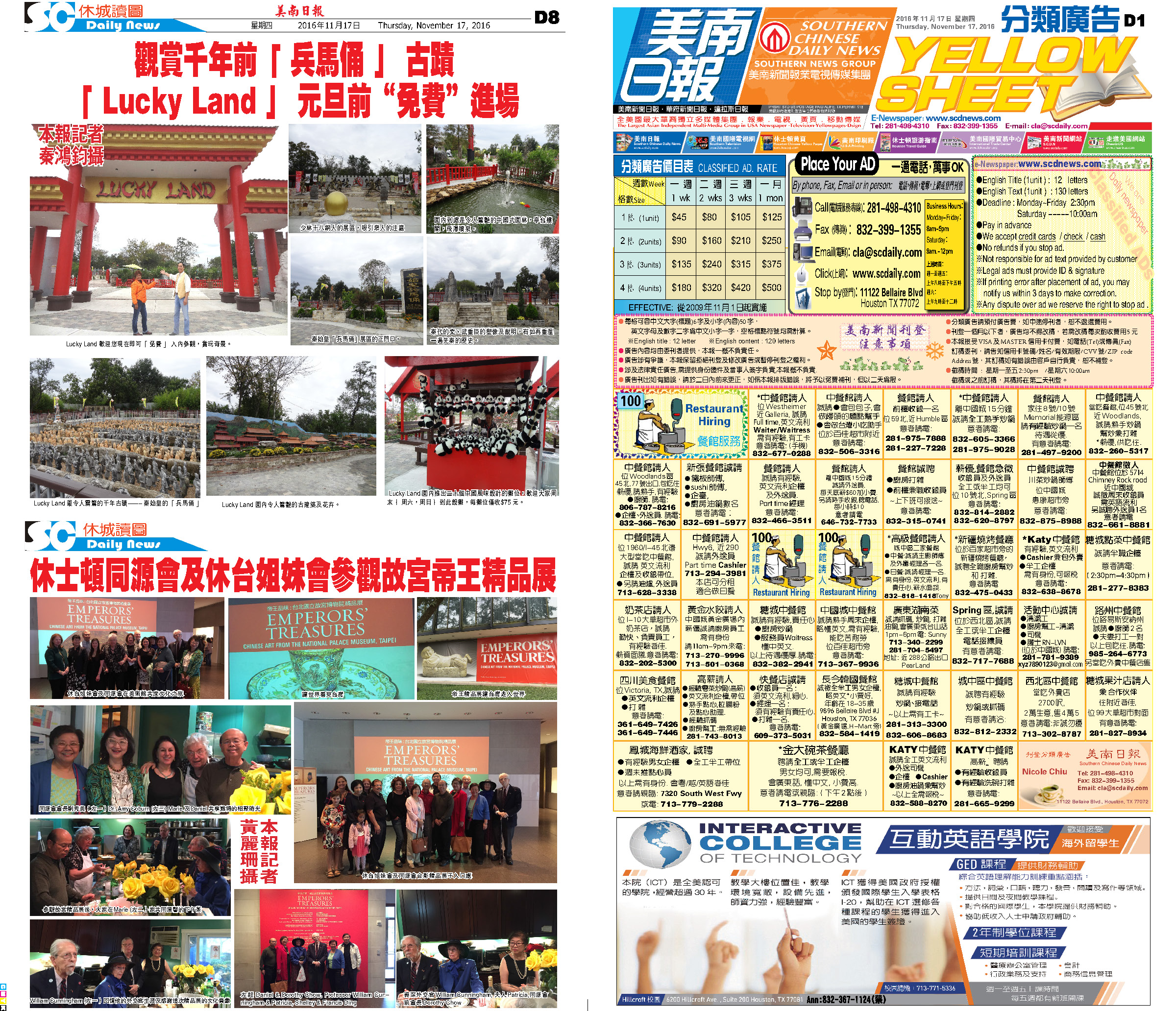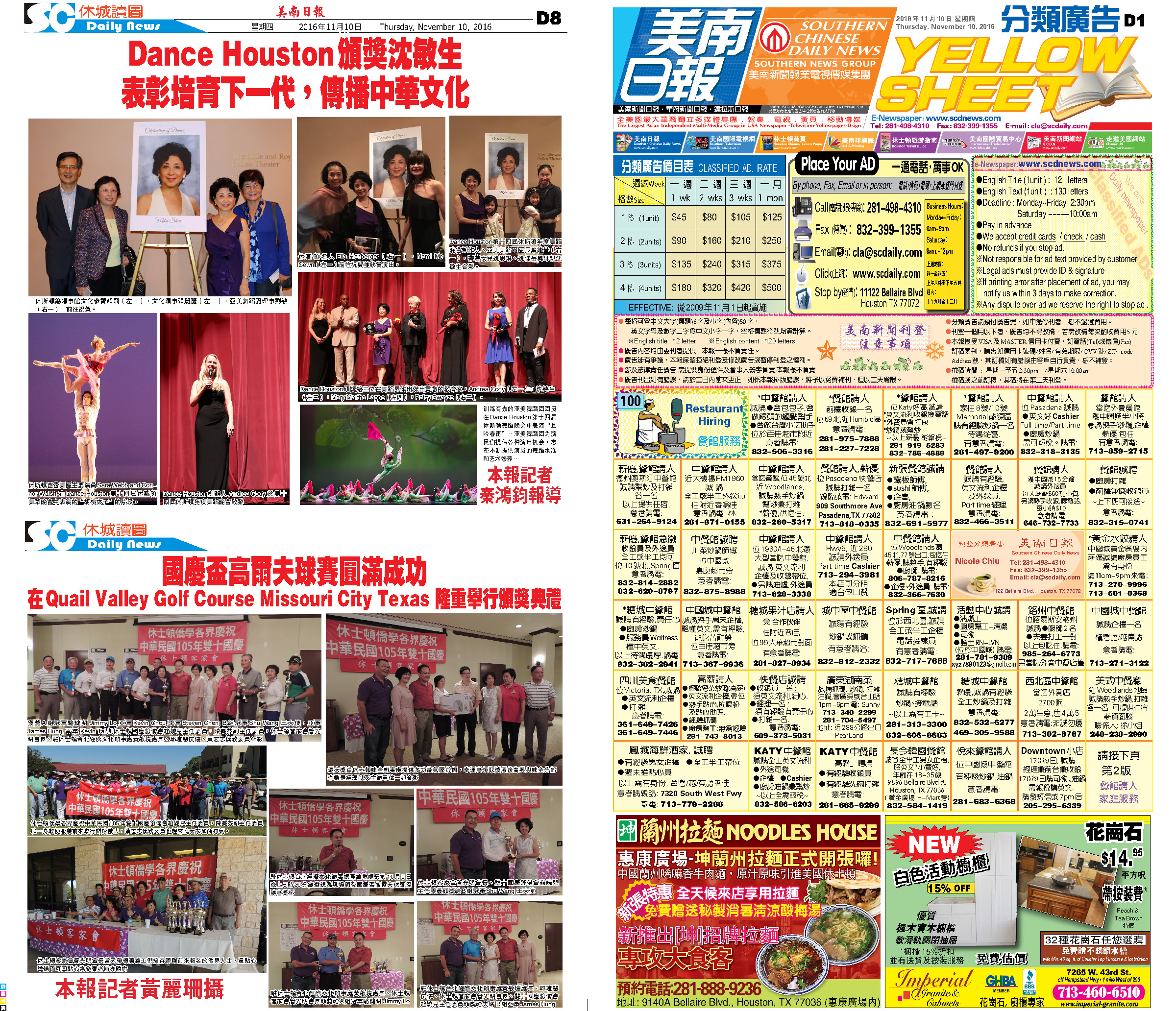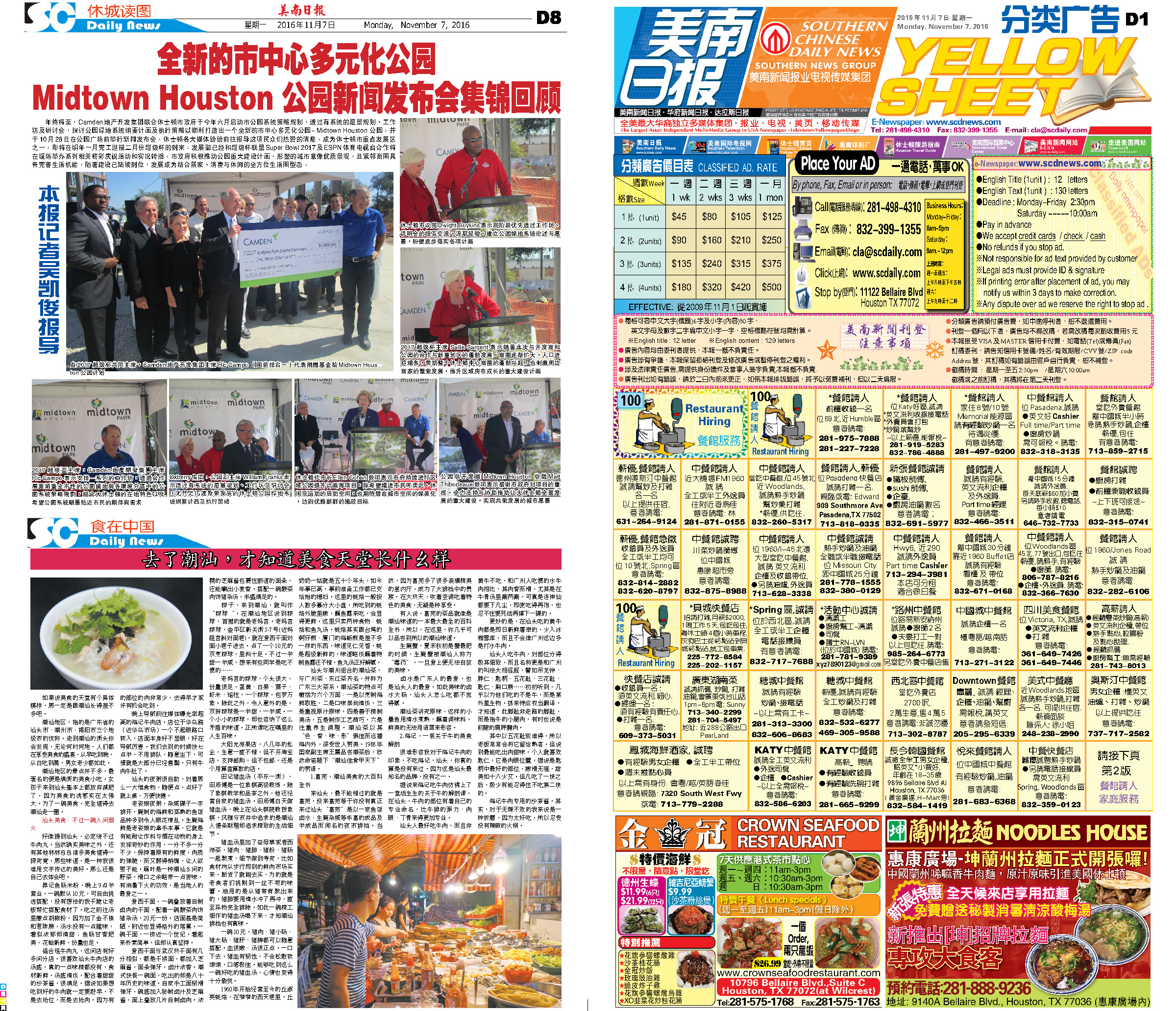161117 Epaper

 A Section B SectionC Section D Section161116 Epaper

 A Section B SectionC Section D Section161115 Epaper

 A Section B SectionC Section D Section161114 Epaper

 A Section B SectionC Section D Section161113 Epaper

 A Section B SectionC Section D Section161112 Epaper

 A Section B SectionC Section D Section161111 Epaper

 A Section B SectionC Section D Section161110 Epaper

 A Section B SectionC Section D Section161108 Epaper

 A Section B SectionC Section D Section161109 Epaper

 A Section B SectionC Section D Section161107 Epaper

 A Section B SectionC Section D Section